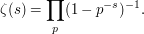## Order by property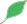This article is a stub.This means that it cannot be considered to contain or lead to any mathematically interesting information.

### Quick description

In order to evaluate some mathematical expression it is often benefitial to combine terms with a common property.

### Prerequisites

Some real analysis.

### Example 1

If one has the Riemann zeta function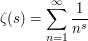then the sum is absolutely convergent for Re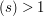.

Therefore it can be rearranged in every possible manner. Now let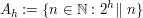where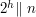means that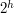dividesbut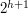does not. Then the set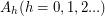form a partition of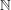and we get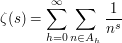But now we can write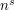as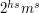wheredoes not divideand we get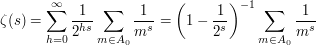by the geometric sum formula. Applying this to all the other primes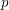and using a limiting argument we establish the product formula